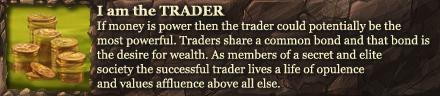# Celtic Heroes

## The Official Forum for Celtic Heroes, the 3D MMORPG for iOS and Android Devices

#1
Hey guys I dare for you to ask Siri what's 0 divide by 0

#4
lvl 1+ Mage on Donn

#5
The weird thing is though, 0 is divisible by 0, the quotient would be 0.
I am a Moderator Need any help? Private message me here or send me a mail in-game, Liviticus on every world.

#6
Liviticus wrote:The weird thing is though, 0 is divisible by 0, the quotient would be 0.

The quotient would be all real numbers. 0x2 is still 0
Houston/rogue216-lvl 216 combined-140 rogue and 76 druid,

Become one with the 216 today! Consultations are Monday- Friday 8 am to 6pm. No walk-ins, only scheduled appointments allowed!

#7
But 0x0=0? My mind has been blown. Next thing you know 10+10=20.
1+1=2
2+2=4
3+3=6
4+4=8
5+5=10
6+6=12
7+7=14
8+8=16
9+9=18
10+10=20
11+11=22
12+12=24
13+13=26
14+14=28
15+15=30
16+16=32
17+17=34
18+18=36
19+19=38
20+20=40
^this right here should help people get past this years math class
Thanks for Cross Platform OTM!

#8
10 divide by 0

#9
Gandorf Biggums wrote:But 0x0=0? My mind has been blown. Next thing you know 10+10=20.
1+1=2
2+2=4
3+3=6
4+4=8
5+5=10
6+6=12
7+7=14
8+8=16
9+9=18
10+10=20
11+11=22
12+12=24
13+13=26
14+14=28
15+15=30
16+16=32
17+17=34
18+18=36
19+19=38
20+20=40
^this right here should help people get past this years math class

This years math class is 1st grade. 800th post ftw

#10
Wow I have no friendsMain account-
Thebeast1221 lvl 127
Chieftain of Violation
Danu
(Thebeast1221 name already taken that's why 1224)
"You won't be getting any thanks from me, now shut you mouth and watch your back''
[CENTER][/CENTER]

### Who is online

Users browsing this forum: No registered users and 14 guests